# Prigogine-Defay ratioThis article is a 'stub' page, it has no, or next to no, content. It is here at the moment to help form part of the structure of SklogWiki. If you add sufficient material to this article then please remove the {{Stub-general}} template from this page.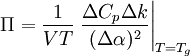$\Pi = \frac{1}{VT} \left. \frac{\Delta C_p \Delta k}{ (\Delta \alpha)^2} \right|_{T=T_g}$
where$V$ is the volume,$T$ is the temperature,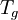$T_g$ is the temperature of the glass transition,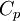$C_p$ is the isobaric heat capacity,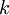$k$ is the compressibility and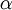$\alpha$ is the thermal expansion coefficient.